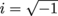# SAT Math: Applying Properties of Imaginary Numbers to Simplify a Complex Fraction

Ifand the complex number above is rewritten as a + bi, where a and b are real number constants, what is the value of a? Round to one decimal place.

 Additional Topics in Math Circle theorems Product Type SAT Math SAT SAT Math SAT Math Additional Topics in MathNumbers and Operations Test Prep SAT Math